# How Many Paths are Possible in an 18 Hole Round of Match Play Golf?

## And a practical investigation of real-life USGA match play data

In honor of the Ryder Cup, here’s a fun puzzle for the mathematically inclined golfer to consider: how many different paths are possible in an 18 hole round of match play golf?

If you’d rather not wade through the math then you can skip ahead to the “practical exploration” section of this post to see some actual match play data, but if you like puzzles then let’s assume the following match play rules, adapted and condensed via the USGA:

• A match consists of one side (individual or team) playing against another over a round of 18 holes
• A hole is won by the side that holes its ball in the fewer strokes
• A hole is halved if each side holes out in the same number of strokes
• A match is won when one side leads by a number of holes greater than the number remaining to be played
• If the match is tied after 18 holes, it ends as a tie

We can depict the set of all possible match play paths as a tree that looks like this: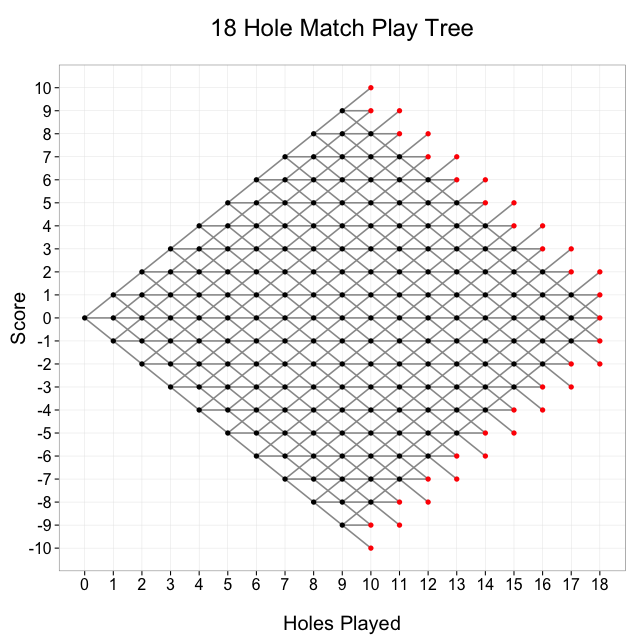This tree shows all possible paths in an 18 hole round of match play. The number of holes played is on the x-axis, and the score of the match is on the y-axis. Every match starts at (0, 0), and moves from left to right. The match ends after 18 holes have been played, or one player’s lead is greater than the number of holes remaining. The black dots represent nodes at which the match continues, and the red dots represent nodes at which the match is over

## How many paths are there across this tree?

Let’s say that Adam and Bubba are playing a match, and we’ll arbitrarily score it from A’s perspective, so that a positive score means that A is winning and a negative score means that B is winning. Every match starts at the leftmost point of the tree: 0 holes played, 0 score (“all square”). The match progresses hole by hole, and moves from left to right across the tree. There are 3 possible outcomes on each hole: A wins the hole, B wins the hole, or the hole is halved. We can denote A winning a hole with an up arrow (↑), B winning a hole with a down arrow (↓), and a halve with a right arrow (→).

Any path can then be written as a sequence of arrows. For example, one path is where the two players halve each hole for 18 consecutive holes, resulting in a tied match:

→ → → → → → → → → → → → → → → → → →

Another path would be if A won the first 10 holes. In that scenario, A would be up by 10 holes with 8 to play, and so the match would be over:

↑ ↑ ↑ ↑ ↑ ↑ ↑ ↑ ↑ ↑

From basic combinatorics, we know there are 318 = 387,420,489 sequences that are 18 characters long and contain only the ↑, →, and ↓ characters. However, many of those sequences are not valid match play paths, because the match ends when one player’s lead is greater than the number of holes to play. For example, the sequence of 18 consecutive ↑ characters is invalid because the match would end after 10 holes. It’s worth noting though that 318 serves as an upper bound on the final answer.

If we wanted to follow this combinatoric approach to the problem, we could do it, but it would be pretty tedious and annoying. We could phrase a series of questions like, “how many character sequences are there that contain only ↑, →, and ↓, are 12 characters long, end with ↑ or →, and contain exactly 7 more ↑ characters than ↓ characters?” That’s an answerable question (3,157), but it only tells us one small piece of the puzzle: the number of possible paths in a match that ends with a score of +7 after 12 holes played, or “7 & 6” in golf terminology (“7 up with 6 to play”). We could answer a question like the one above for every possible terminal node, then sum the results, but maybe there’s a (slightly) more elegant way to do this?

## Work backward!

Backward induction provides a much-needed respite from the complication of combinations and permutations. Instead of trying to construct sequences of arrows subject to multiple constraints, we can work across the tree backward, i.e., from right to left, defining the number of paths to a node in terms of the number of paths to the nodes before it.

Let’s simplify the problem a bit by looking at the tree for a 3 hole match: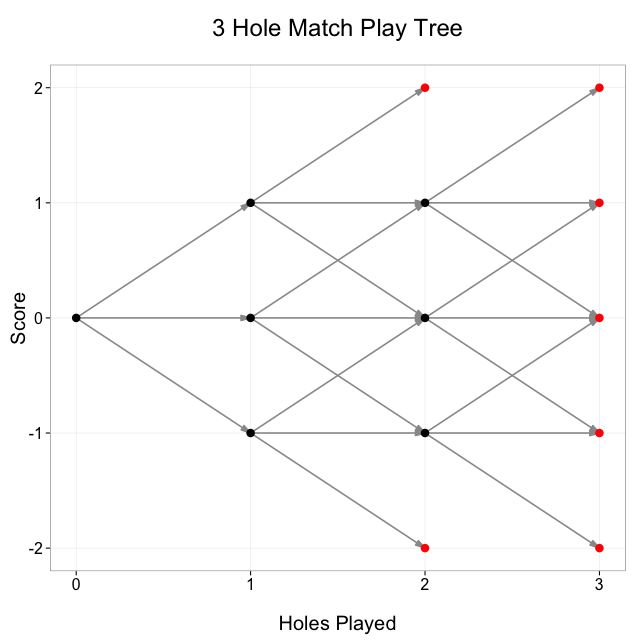Take a look at the node in the tree at (2, 0): that’s 2 holes played, 0 score. How could we have gotten to that point? Well, we can look at all the line segments coming into the node and see that there were 3 possible previous states: (1, 1), (1, 0), and (1, -1), so the number of paths to (2, 0) is equal to the sum of the number of paths to each of (1, 1), (1, 0), and (1, -1).

Now look at the node at (3, 1): there are only 2 possible previous nodes, (2, 1) and (2, 0). A 3 hole match could not have been at (2, 2) before coming to (3, 1) because (2, 2) is a terminal node so the match would have ended there. Other nodes, for example (1, 1), have only 1 possible previous node.

So every node after (0, 0) has exactly 3, 2, or 1 possible previous nodes, and we can define the number of paths to a given node as the sum of the number of paths to its possible previous nodes. (0, 0) is a special base case because every match starts there, so we can say that there is exactly 1 path that gets a match to (0, 0). Let p(h, s) equal the number of paths to the node at h holes played, and score s. Then our full induction specification looks like this: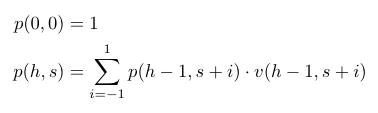Where v(h, s) is a boolean function whose value is 1 if (h, s) is a valid node where the match continues, and 0 otherwise: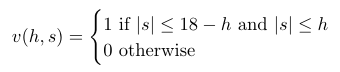For example, v(10, 10) = 0 because the match does not continue if the score is +10 after 10 holes, v(0, 1) = 0 because a score of +1 after 0 holes is not a valid score, and v(1, 1) = 1 because +1 after 1 hole is a valid node and the match continues.

With the above induction specification, the final step is to evaluate p(h, s) for every possible terminal node in the 18 hole tree (i.e. all the red nodes), then take the sum to calculate the total number of possible paths. I wrote an R script to do this, which you can see on GitHub. The output gives a final answer of 169,688,089 total possible paths. This is less than half of the 318 number we hypothesized earlier as an upper bound. 132,458,427 of the valid paths are 18 characters long, which means that 34.2% of all 18 character sequences of ↑, →, and ↓ characters are valid paths. As a sanity check I also did the backward induction in a Google spreadsheet, which got the same answer and makes for a nice visual aid.

## A practical exploration of actual USGA match play data

Now that we know there are roughly 170 million possible match play paths, we can turn to actual data to see what the real-life distribution of paths looks like. The Ryder Cup, USGA amateur tournaments, and WGC-Accenture Match Play Championship all use a match play format. The USGA data was the most accessible so I wrote a quick Ruby script to scrape hole-by-hole scores from every USGA amateur match from 2010 through 2014—a total of 50,773 holes played over 3,112 matches—and dump the results into a .csv file.

Intuitively we shouldn’t expect every path to occur with equal probability. The paths would have equal probability if every hole were an independent event with 1/3 probability for each of ↑, →, and ↓, but the holes are not independent, and the probabilities are not all 1/3. In fact of all the holes played in the dataset, 42% resulted in a halve, with the other 58% won by one of the players. Since a halve occurs more than 1/3 of the time, that will tend to put more weight on the paths that stay closer to 0 score as opposed to larger magnitude scores.

On the other hand, each hole is not an independent event. Even though USGA championships are selected to include only highly skilled players, there will inevitably be some matches where one player is better than the other, and these matches will tend to have larger margins of victory. It would seem particularly unlikely, for example, to see a path where A wins each of the first 9 holes then B wins each of the second 9 holes, resulting in a tie. If A won 9 consecutive holes, it’d be a pretty safe bet that A is the better player than B, and very unlikely that B would win 9 consecutive holes against the superior player.

Of the 3,112 USGA matches that I scraped, there are 3,110 unique paths. There are two paths that appear twice each, one that finishes 8 & 7, the other 4 & 3. The overall distribution of final scores from the USGA matches is wider and flatter than the distribution would be if we picked paths randomly from a uniform distribution: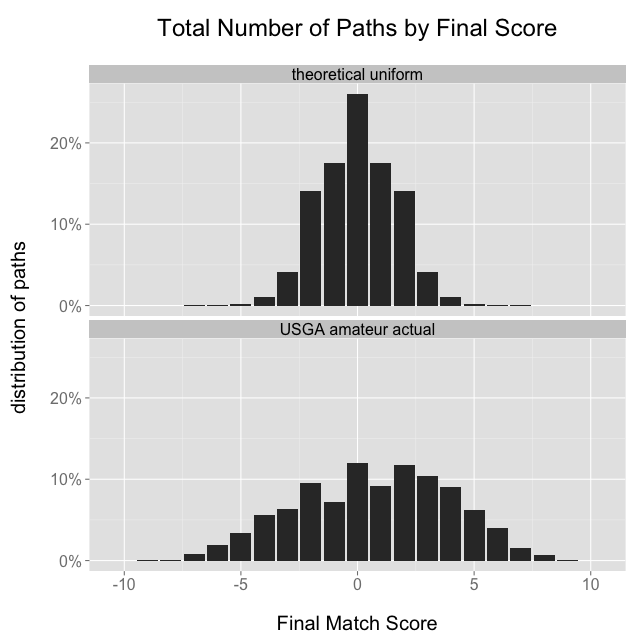This shouldn’t be too surprising, because as mentioned earlier the theoretical uniform distribution would come true if every hole were an independent event with equal probabilities for ↑, →, and ↓, and we don’t believe that to be the case. The wider actual distribution might suggest that many matches are between players of unequal ability: if some players are better than others then we would expect the flatter distribution with more large margins of victory.

We can also investigate whether the likelihood of winning the next hole is a function of the current score: all things held constant, we should probably expect the currently leading player to be the better player, and therefore more likely to win the next hole. I took all back-9 holes and aggregated the probabilities for winning, losing, and halving the next hole given the current match score, and sure enough the probability of a player winning a hole increases as a function of that player’s lead: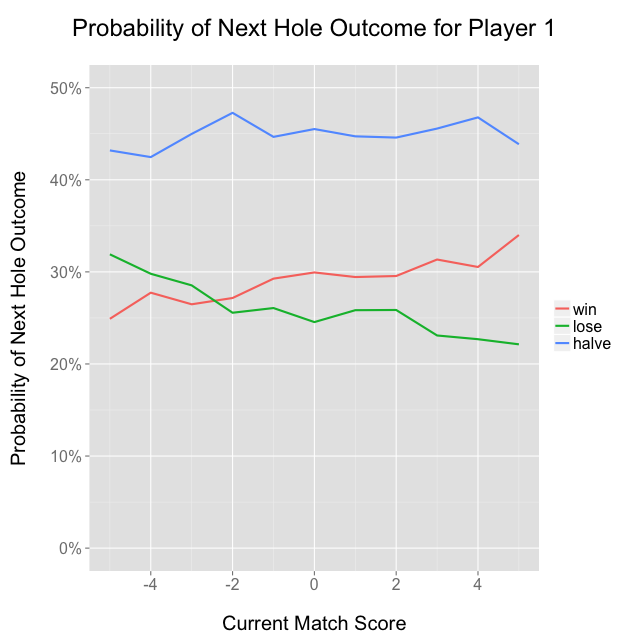It’s a bit strange that the seemingly arbitrary “player 1” seems to win more than average, but I think it’s probably because USGA tournaments are seeded based on qualifying stroke play scores, and “player 1” as I’ve defined it is the stronger seed, at least in the early rounds. “Player 1” won 60.3% of the 3,112 matches, which would be an extremely unlikely result if each match were independent with 50/50 odds, so that might suggest the ordering of player names on the USGA’s website is somehow related to skill level.

Another potentially interesting phenomenon is the relative paucity of matches that end with a score of ±1 as opposed to 0 or ±2. I have no supporting data, but I’d hypothesize that players who are down 1 on the final hole tend to play more aggressively, which makes them more likely to either win or lose the hole, and less likely to halve it—imagine an aggressive birdie putt, which maybe goes in, or maybe goes 6 feet past the hole and leads to a bogey. It’s also possible that the effect is just noise and I’m making up a story about it, which is always an important caveat!

## Kind of like that Facebook map

Finally, I thought it’d be fun to build something like the Facebook friendship map, except with the match play tree. The brightness and thickness of each segment represents the number of USGA matches that passed through that segment, so for example the segment from (0, 0) to (1, 0) is the brightest because that’s the most common path: every match starts at (0, 0), and more of them move to (1, 0) than any other node.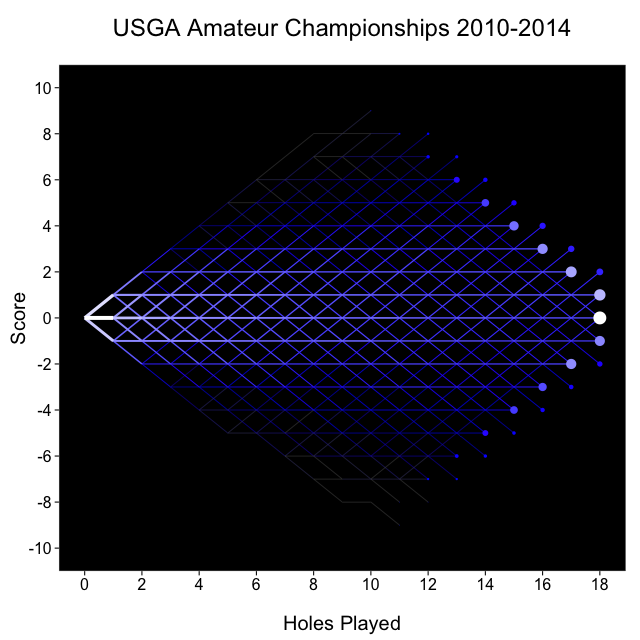## Data and code on GitHub

The USGA hole-by-hole data is available as a .csv at https://github.com/toddwschneider/matchplay, along with R code to calculate the number of paths, draw match play trees, and do some data analysis, plus Ruby code to scrape the data. There’s also a Google spreadsheet with the number of paths calculation.